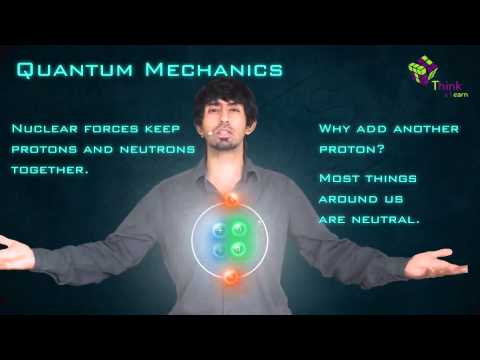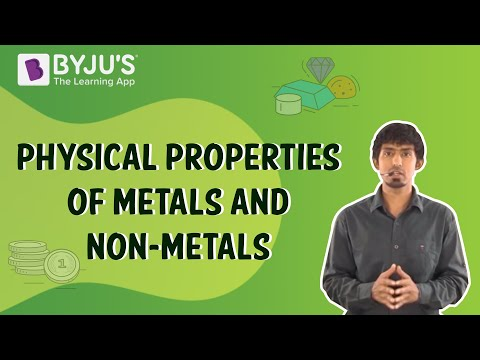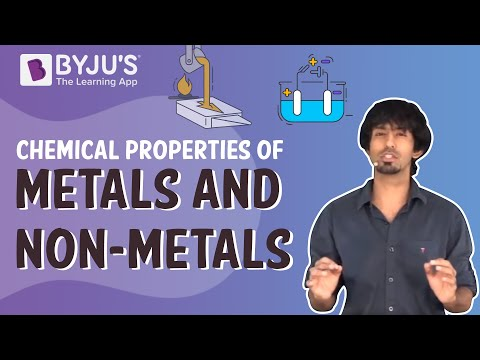# Metals and Nonmetals Questions

We are surrounded by a plethora of materials. Each material has a distinguishing property that sets it apart from the others. Some materials are hard, while others are soft, and some quickly break while others do not. Some materials are better at carrying electricity and heat than others.

There is a class of materials that conduct heat well, can carry electricity, and are often heavy and difficult to break into little pieces. This category of materials is called Metals. For example – Aluminum, Copper, Lead, Gold, Silver, etc.

There is another group that is just the immediate opposite of metals and they are called Non-metals. For example – Wood, glass, plastic, etc.

 Definition: Metals and nonmetals are two categories of materials that we come into contact with on a daily basis. Metals and nonmetals are the two types of elements, and it’s crucial to know which one is which. Nonmetals (such as phosphorus and sulphur) are insulators, whereas metals (such as copper and aluminium) are good conductors of heat and electricity. The properties of a material are used to classify it.

## Metals and Nonmetals Chemistry Questions with Solutions

Q1: Give the physical and chemical properties of metals and non-metals.

The following are some of the physical properties of metals:

• Nature is lustrous (shiny).
• Metal is an excellent heat and electrical conductor.
• The melting point and density are both high.
• Ductile
• Mouldable (Malleable)
• Except for mercury, it is solid at room temperature.
• Opaque

The following are some of the chemical properties of metals:

• Easy to corrode
• Electrons can be lost.
• Develop basic oxides
• Have low electronegativities
• Good reducing agents

Some physical properties of non-metals are as follows:

• Poor heat and electricity conductors
• Non-Ductile metals
• Solids that are brittle
• Maybe solids, liquids or gases at room temperature
• These aren’t sonorous at all.
• Transparent

The chemical properties of non-metals are:

• The number of electrons in the outer shell is usually 4-8
• Conveniently gain or lose valence electrons
• When they come into contact with oxygen, they produce acidic oxides.
• High electronegative elements
• Excellent oxidising agents

Q2: Write one example of each of

(i) a metal that is so soft that, it can be cut with a knife and a non-metal which is the hardest substance.

(ii) metal and non-metal which exist as a liquid at room temperature.

(i) Sodium, carbon (diamond).

(ii) Mercury is a liquid metal, and bromine is a liquid non-metal.

Q3: Mention the names of the metals for the following:

(i) Two metals that are alloyed with iron to make stainless steel.

(ii) Two metals which are used to make jewellery.

(i) Nickel and chromium.

(ii) Gold and platinum.

Q4: Write the difference between metals and non-metals.

For chemists, a reactivity series is an essential tool. It assists us in comprehending the properties of metals and their distinctions.

Metals

Non-metals

Except for mercury, these are solids at room temperature.

These can be found in all three states.

Except for sodium, they are very hard.

Except for diamonds, these are all soft.

These are malleable and ductile

These are brittle and can break down into pieces

These are shiny

These are non-lustrous except iodine

Electropositive in nature

Electronegative in nature

Have high densities

Have low densities

Q5: The most abundant metal in the earth’s crust is

(a) Iron

(b) Calcium

(c) Aluminium

(d) Sodium

Q6: The poorest conductor of heat among metals is

(a) Mercury

(c) Calcium

(d) Sodium

Q7: Which property of metals is used for making bells and strings of musical instruments like Sitar and Violin?

(a) Ductility

(b) Malleability

(c) Sonorousness

(d) Conductivity

Q8: Al2O3 + 2NaOH → …… + H2O

(a) Al(OH)3

(b) Na2O

(c) NaAlO2

(d) AlNaO2

Q9: Which of the following is the correct arrangement of the given metals in ascending order of their reactivity?

Zinc, Iron, Magnesium, Sodium

(a) Zinc > Iron > Magnesium > Sodium

(b) Sodium > Magnesium > Zinc > Iron

(c) Sodium > Zinc > Magnesium > Iron

(d) Sodium > Magnesium > Iron > Zinc

Answer: (b) Sodium > Magnesium > Zinc > Iron

Q10: The process of converting a carbonate ore into metal oxide by rapidly heating it in the absence of air is known as

(a) Roasting

(b) Reduction

(c) Smelting

(d) Calcination

Q11: You are given samples of three metals. Sodium, magnesium, and copper. Recommend any two tasks to arrange them in order of decreasing activity.

Sodium forms sodium hydroxide and hydrogen gas when it reacts vigorously with cold water.

$$\begin{array}{l}2Na(s) + 2H_{2}O(l) \to 2NaOH(aq) + H_{2}(g)\end{array}$$

Magnesium forms magnesium hydroxide and hydrogen gas when it reacts with hot water rather than cold water.

$$\begin{array}{l}Mg(s) + 2H_{2}O(l) \to Mg(OH)_{2}(aq) + H_{2}(g)\end{array}$$

Hence sodium is more reactive than magnesium.

Q12: Suggest a procedure of reduction for the following metals during their metallurgical processes:

(i) metal ‘A’ which is one of the last, second or third positions in the reactivity.

(ii) metal ‘B’ which gives a vigorous reaction even with water and air.

(iii) metal ‘C’ which is kept in the middle df activity series.

(i) Chemical reduction using carbon or carbon monoxide as a reducing agent can provide ‘A.’

(ii) Electrolytic reduction can be used to obtain ‘B.’

(iii) Reducing agent, such as ‘Al,’ can be used to reduce the value of ‘C.’

Q13: Mention the general properties of Non-metals.

High ionisation energy and electronegativity are two features of non-metals. Non-metals usually gain electrons when reacting with other compounds, forming covalent bonds, due to their features. The anionic dopants have a strong influence on the VB among the non-metals. Carbon, nitrogen, fluorine, sulphur, and iodine are non-metal dopants.

Non-metals have the following properties in general:

1. Non-metal atoms are usually smaller than metal atoms. The atomic sizes of non-metals determine the number of additional properties.
2. Electrical conductivities of non-metals are extremely low. The most important property that differentiates non-metals from metals is their low or non-existent electrical conductivity.
3. Electronegativities are high in non-metals. This means that non-metal atoms have a significant tendency to attract more electrons than they would otherwise have.
4. Electronegativities in non-metals are high. This means that non-metal atoms have a significant desire to keep their existing electrons. Metals, on the other hand, readily give away one or more electrons to non-metals, resulting in the formation of positively charged ions and the conductivity of metals.
5. Some non-metals exist as gases, while others exist as solids, and one exists as a liquid under normal conditions of temperature and pressure. At room temperature, all metals except mercury are solids. Since many non-metals are liquids or gases, their melting and boiling points are often low under normal atmospheric circumstances.
6. Non-metals have a tendency to be fragile in their solid state. As a result, they lack the malleability and ductility that metals have.

Q14: (a) Give the chemical name of the coating that develops on silver and copper articles when these are left exposed to moist air.

(b) Describe what is galvanisation. What purpose would it serve?

(c) Define the term alloy. What is the process for making alloys? What happens to the properties of iron when:

(i) small quantity of carbon is mixed with it

(ii) nickel and chromium are mixed with it.

(a) Ag2S (silver sulphide) is created on silver, basic copper carbonate CuCO3. On copper, CU(OH)2 is formed.

(b) Galvanisation is the method of coating zinc to iron. It is used to prevent iron from rusting.

(c) A homogeneous combination of two or more metals is known as an alloy. One of them could be non-metal. Melting two or more metals together creates alloys.

(i) When a small amount of carbon is added to iron, it does not rust.

(ii) When we create an alloy of iron with nickel and chromium, we receive stainless steel which is malleable and could not get rusted.

Q15: Briefly describe the chemical properties of Metals.

Metals are electropositive in nature, which donate electrons and form positive ions, allowing them to become stable. Consider the following scenario:

Na → Na+ + e

Some of the chemical properties of metals are as follows:

Reaction of Metals with Oxygen (of air)

• When metals are burnt in air, metals combine with oxygen to produce metal oxides.

Metals + Oxygen (from air) → Metal Oxide (basic oxide)

Example: At room temperature, sodium metal reacts with oxygen in the air to form sodium oxide, a basic oxide:

$$\begin{array}{l}4Na(s) + O_{2}(g) \to 2Na_{2}O(s) \end{array}$$

• Alkalis are formed when metal oxides dissolve in water. For example, sodium oxide, a basic oxide, reacts with water to produce sodium hydroxide, an alkali:

$$\begin{array}{l}Na_{2}O(s) + H_{2}O(l) \to 2NaOH(aq)\end{array}$$

• Magnesium, on the other hand, does not react with oxygen at room temperature. However, when magnesium is heated, it burns in the air, producing extreme heat and light, resulting in the formation of a basic oxide called magnesium oxide, which is a white powder:

$$\begin{array}{l}2Mg(s) + O_{2}(g) \to 2MgO(s)\end{array}$$

• Even when heated to extreme temperatures, iron metal does not burn in the air. When iron reacts with oxygen, it produces iron (II, III) oxide:

$$\begin{array}{l}3Fe(s) + 2O_{2}(g) \to Fe_{3}O_{4}(s)\end{array}$$

Reaction of Metals with Water

• Some metals produce metal hydroxide when they react with water, while others do not. The reactivity of metals with water varies.

Metal hydroxide and hydrogen gas are the products created when a metal combines with water (cold or hot water):

Metal + Water → Metal hydroxide + Hydrogen

• Potassium forms potassium hydroxide and hydrogen gas when it reacts vigorously with cold water:

$$\begin{array}{l}2K(s) + 2H_{2}O(l) \to 2KOH(aq) + H_{2}(g) + Heat\end{array}$$

• Coldwater has no effect on magnesium metal. Magnesium forms magnesium hydroxide and hydrogen when it reacts with hot water:

$$\begin{array}{l}Mg(s) + 2H_{2}O(l) \to Mg(OH)_{2}(aq) + H_{2}(g)\end{array}$$

• When aluminium reacts with steam, aluminium oxide and hydrogen gas are formed:

$$\begin{array}{l}2Al(s) + 3H_{2}O(g) \to Al_{2}O_{3}(s) + 3H_{2}(g)\end{array}$$

Reaction of Metals with Dilute Acids

• A metal salt and hydrogen gas are produced when a metal combines with a dilute acid:

Metal + Dilute acid → Metal salt + Hydrogen

• When sodium metal reacts with dilute hydrochloric acid, sodium chloride and hydrogen are formed:

$$\begin{array}{l}2Na(s) + 2HCl(aq) \to 2NaCl(aq) + H_{2}(g)\end{array}$$

Reaction of Metals with Salt Solutions

• When a more reactive metal is added to a less reactive metal’s salt solution, the less reactive metal is displaced from the salt solution by the more reactive metal.

Salt solution of metal B + Metal A → Salt solution of metal A + Metal B

• The Reaction of Zinc with Copper Sulphate Solution. Copper sulphate is a dark blue liquid. When a strip of zinc metal is dipped in copper sulphate solution, the blue colour fades over time as a colourless zinc sulphate solution forms, resulting in the deposition of red-brown copper metal on the zinc strip:

$$\begin{array}{l}CuSO_{4}(aq) + Zn(s) \to ZnSO_{4}(aq) + Cu(s)\end{array}$$

When a strip of copper metal is placed in a zinc sulphate solution, however, there is no reaction.

## Practise Questions on Metals and Nonmetals

Q1: Which of the following pairs will give displacement reactions?

(a) FeSO4 solution and Copper metal

(b) CuSO4 solution and Silver metal

(c) AgNO3 solution and Copper metal

(d) NaCl solution and Copper metal

Q2: Non-metals form covalent chlorides because

(a) they can give electrons to chlorine

(b) they cannot share electrons with chlorine atoms

(c) they can give electrons to chlorine atoms to form chloride ions

(d) they can share electrons with chlorine

Q3: Give reason for the following:

(i) Metals are used to make school bells.

(ii) Copper is used to make electric wires.

Q4: Define the terms:

(i) mineral

(ii) ore, and

(iii) gangue.

Q5: Give reasons for the following:

(i) When silver and copper are exposed to air, they lose their shine. In each case, identify the substance that has formed on its surface.

(ii) Tamarind juice is used to clean tarnished copper objects.

(iii) Although aluminium is more reactive than iron, it exhibits less corrosion when exposed to air when compared to iron.

Click the PDF to check the answers for Practice Questions.

## Recommended Videos

#### Metals and Non-metals Introduction#### Physical Properties of Metals and Non-metals#### Chemical Properties of Metals and Non-metals